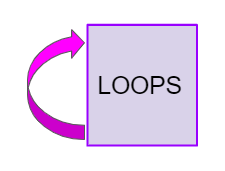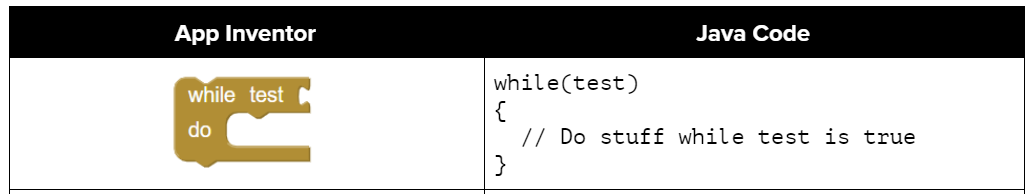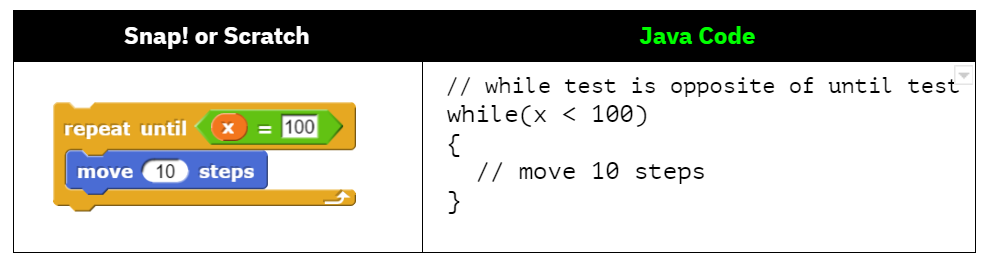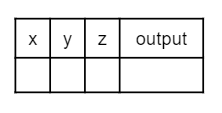# 4.1. While Loops¶When you play a song, you can set it to loop, which means that when it reaches the end it starts over at the beginning. A loop in programming, also called iteration or repetition, is a way to repeat one or more statements. If you didn’t have loops to allow you to repeat code, your programs would get very long very quickly! Using a sequence of code, selection (ifs), and repetition (loops), the control structures in programming, you can construct an algorithm to solve almost any programming problem!

A while loop executes the body of the loop as long as (or while) a Boolean condition is true. When the condition is false, we exit the loop and continue with the statements that are after the body of the while loop. If the condition is false the first time you check it, the body of the loop will not execute.

Notice the while statement looks a lot like an if statement, but it runs more than once. The curly brackets { } are optional when there is just 1 statement following the condition, but it is a good idea to put them in anyway because loops often have more than 1 statement in the body.

// if statements just run once if the condition is true
if (condition)
{
statements;
}
// while statements are repeated while the condition is true
while (condition)
{
statements;
}


If you took AP CSP with a block programming language like App Inventor, you may have used a loop block like below that looks very similar to Java while loops (or you may have used a for loop which will be covered in the next lesson). Almost every programming language has a while loop.Figure 1: Comparing App Inventor and Java for while loops

If you’re used to a language like Snap! or Scratch, you may be familiar with the Repeat Until loop. However, you have to be very careful comparing repeat until to while loops. The while test is the opposite of the repeat until test. For example, if you are repeatedly moving until reaching x position 100, you must create a Java while loop that repeatedly moves while it has not yet reached x position 100 or is less than 100 as below.Figure 2: Comparing Snap! or Scratch Repeat Until Loop to Java while loop

Here’s what the flow of control looks like in a Java while loop. Notice that while the condition is true, the loop body is repeated.

The loop condition usually involves a loop control variable that controls when to stop the loop. The simplest loops are counter-controlled loops like below, where the loop variable is a counter that controls how many times to repeat the loop. There are 3 steps to writing a loop using this loop control variable as seen below in a loop that counts from 1 to 10.

Note

Remember these 3 steps to writing a loop:

1. Initialize the loop variable (before the while loop)

2. Test the loop variable (in the loop header)

3. Change the loop variable (in the while loop body at the end)

Here is a while loop that counts from 1 to 5 that demonstrates the 3 steps of writing a loop. Can you change it to count from 2 to 10? Can you make it count by 2s? Can you make it count backwards?

Java doesn’t require your code to be correctly indented (code moved to the right a few spaces) to make it clear what statements are part of the body of the loop, but it is standard practice to do so.

Note

On the free response part of the exam, the reader will use the indention when determining the meaning of your code, even if you forget the open or close curly brace.Check your understanding

4-1-1: Consider the following code segment. Which of the following can be used as a replacement for the missing loop header so that the loop prints out “0 2 4 6 8 10”?

int count = 0;
{
System.out.print(count + " ");
count += 2;
}

• while (count == 10)
• This would not print out anything because count = 0 at the start of the loop, so it never equals 10.
• while (count < 10)
• This would print out 0 2 4 6 8. Try it in the Active Code window above.
• while (count <= 10)
• Yes, try it in the Active Code window above.
• while (count > 10)
• This would not print out anything because count = 0 at the start of the loop, so it is not greater than 10.

## 4.1.1. Tracing Loops¶

A really important skill to develop is the ability to trace the values of variables and how they change during each iteration of a loop.

You can create a tracing table that keeps track of the variable values each time through the loop as shown below. This is very helpful on the exam. Studies have shown that students who create tables like this do much better on code tracing problems on multiple choice exams.Figure 5: A trace table showing the values of all of the variables each time through the loop. Iteration 0 means before the loop.

Watch the following video for a tracing demo. When you are tracing through code, pretend to be the computer running the code line by line, repeating the code in the loop, and keeping track of the variable values and output.

Video: (TZss5ukwN8s)Check your understanding

4-1-2: Consider the following code segment. What is count’s value after running this code segment? (To trace through the code, keep track of the variable count and its value through each iteration of the loop.)

int count = 1;
while (count <= 10)
{
count *= 2;
}
count = count - 10;

• 0
• Count is changed inside the loop and after the loop.
• 1
• Count is changed inside the loop and after the loop.
• 16
• Don't forget to subtract 10 from count after the loop.
• 6
• Yes, the loop will keep multiplying count by 2 to get 2, 4, 8, 16 and then it subtracts 10 from 16 after the loop.

Step through the code above with the visualizer.

4-1-3: What does the following code print? (To trace through the code, keep track of the variable x and its value, the iteration of the loop, and the output every time through the loop.)

int x = -5;
while (x < 0)
{
x++;
System.out.print(x + " ");
}

• 5 4 3 2 1
• x is initialized (set) to -5 to start.
• -5 -4 -3 -2 -1
• x is incremented (x++) before the print statement executes.
• -4 -3 -2 -1 0
• x is set to -5 to start but then incremented by 1 so it first prints -4.

## 4.1.2. Common Errors with Loops¶

One common error with loops is infinite loops. An infinite loop is one that never stops (the condition is always true).

// an infinite loop
while (true)
{
System.out.println("This is a loop that never ends");
}


The infinite loop above is pretty obvious. But, most infinite loops are accidental. They usually occur because you forget to change the loop variable in the loop (step 3 of a loop).

Another common error with loops is an off-by-one error where the loop runs one too many or one too few times. This is usually a problem with step 2 the test condition and using the incorrect relational operator < or <=.

The while loop should print out the numbers 1 to 8, but it has 2 errors that cause an infinite loop and an off-by-one error. Can you fix the errors? If you run an infinite loop, you may need to refresh the page to stop it (so make sure all active code windows on the page are saved).

## 4.1.3. Input-Controlled Loops¶

You can use a while loop to repeat the body of the loop a certain number of times as shown above. However, a while loop is typically used when you don’t know how many times the loop will execute. It is often used for a input-controlled loop where the user’s input indicates when to stop. For example, in the Magpie chatbot lab on repl.it below, the while loop stops when you type in “Bye”. The stopping value is often called the sentinel value for the loop. Notice that if you type in “Bye” right away, the loop will never run. If the loop condition evaluates to false initially, the loop body is not executed at all. Another way to stop the loop prematurely is to put in a return statement that makes it immediately return from the method.

Here’s another example with numbers on repl.it. This code calculates the average of positive numbers, but it is missing the condition for the loop on line 14. Let’s use -1 as the sentinel value. Add the condition to the while loop to run while the user does not input -1. What would happen if you forgot step 3 (change the loop variable - get a new input)? Try commenting out line 19 with // to see what happens (note there is a stop button at the top!).

There are standard algorithms that use loops to compute the sum or average like above, or determine the minimum or maximum value entered, or the frequency of a certain condition. You can also use loops to identify if some integers are evenly divisible by other integers or identify the individual digits in an integer. We will see a lot more of these algorithms in Unit 6 with loops and arrays.

## 4.1.4.Programming Challenge : Guessing Game¶We encourage you to work in pairs on this guessing game. In the guessing game, the computer picks a random number from 0-100 and you have to guess it. After each guess, the computer will give you clues like “Too high” or “Too low”. Here’s the pseudocode for the guessing game. Pseudocode is an English description or plan of what your code will do step by step. What’s the loop variable for this program? Can you identify the 3 steps of writing this loop with respect to the loop variable?

1. Choose a random number from 0-100

2. Get the first guess

3. Loop while the guess does not equal the random number,

• If the guess is less than the random number, print out “Too low!”

• If the guess is greater than the random number, print out “Too high!”

• Get a new guess (save it into the same variable)

4. Print out something like “You got it!”

As an extension to this project, you can add a counter variable to count how many guesses the user took and print it out when they guess correctly.

When you finish and run your program, what is a good guessing strategy for guessing a number between 0 and 100? What was your first guess? One great strategy is to always split the guessing space into two and eliminating half, so guessing 50 for the first guess. This is called a divide and conquer or binary search algorithm. If your guess is between 0-100, you should be able to guess the number within 7 guesses. Another extension is to test whether the user got it in 7 guesses or less and provide feedback on how well they did.

For this project, you will need to use the Scanner class for input and repl.it or another IDE of your choice.

4-1-4: After you complete your code on repl, paste in a link to it (click on share) here.

## 4.1.5. Summary¶

• Iteration statements (loops) change the flow of control by repeating a set of statements zero or more times until a condition is met.

• Loops often have a loop control variable that is used in the boolean condition of the loop. Remember the 3 steps of writing a loop:

• Initialize the loop variable

• Test the loop variable

• Change the loop variable

• In loops, the Boolean expression is evaluated before each iteration of the loop body, including the first. When the expression evaluates to true, the loop body is executed. This continues until the expression evaluates to false which signals to exit the loop. If the Boolean expression evaluates to false initially, the loop body is not executed at all.

• A loop is an infinite loop when the Boolean expression always evaluates to true so that the loop never ends.

• Off by one errors occur when the iteration statement loops one time too many or one time too few.

• If the Boolean expression evaluates to false initially, the loop body is not executed at all.

• Input-controlled loops often use a sentinel value that is input by the user like “bye” or -1 as the condition for the loop to stop. Input-controlled loops are not on the AP CS A exam, but are very useful to accept data from the user.

• There are standard algorithms to compute a sum or average.